# WA Discrete Wavelet Transform VI

LabVIEW 2014 Advanced Signal Processing Toolkit Help

Edition Date: June 2014

Part Number: 372656C-01

»View Product InfoDownload Help (Windows Only)

Owning Palette: Discrete Wavelet VIs

Computes the multi-level discrete wavelet transform (DWT) of signal. This VI returns the approximation coefficients at the largest level and the detail coefficients at all levels for a 1D signal input. This VI returns the approximation coefficients and the detail coefficients at all levels for a 2D signal input. This VI computes the DWT at each level by using the lowpass analysis filters, the highpass analysis filters, and a decimation factor of 2. Wire data to the signal input to determine the polymorphic instance to use or manually select the instance.

Use the pull-down menu to select an instance of this VI.

 Select an instance WA 1D Discrete Wavelet Transform (Waveform)WA 1D Discrete Wavelet Transform (Array)WA 2D Discrete Wavelet Transform

## WA 1D Discrete Wavelet Transform (Waveform)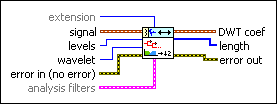extension specifies the method to use to pad data at the borders of the input signal. The extension length is equal to the length of the wavelet filters. When you select the extension method, make the transition between the input signal and the padded data as smooth as possible because a smooth transition generates fewer large detail coefficients and enhances the efficiency of the signal representation.

 0 Zero padding—Uses zeros to pad the input data. Watch for abrupt transitions between the padded zeros and the input data, which causes large artifacts near the transition. 1 Symmetric (default)—Uses replications of the input data to pad the data, except that this VI left-flips the block at the input and right-flips the block at the end. 2 Periodic—Adds a replication of the input data block before and another replication after the input data block to pad the data.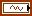signal specifies the input signal.levels specifies the number of levels in the discrete wavelet analysis. levels must be a positive integer no greater than log2(Ls), where Ls is the length of the 1D signal or the minimum dimensional size of the 2D signal. Otherwise, you can set the value to –1, which indicates that this VI sets levels as the largest integer no greater than log2(Ls). The default is –1.wavelet specifies the wavelet type to use for the discrete wavelet analysis. The default is db02. The options include two types: orthogonal (Haar, Daubechies (dbxx), Coiflets (coifx), Symmlets (symx)) and biorthogonal (Biorthogonal (biorx_x), including FBI (bior4_4 (FBI))), where x indicates the order of the wavelet. The higher the order, the smoother the wavelet. The orthogonal wavelets are not redundant and are suitable for signal or image denoising and compression. The biorthogonal wavelets usually have the linear phase property and are suitable for signal or image feature extraction. If you want to use other types of wavelets, do not wire this input. Instead, use the Wavelet Design Express VI to design the wavelet you want and wire the resulting analysis filters to the analysis filters input.error in describes error conditions that occur before this node runs. This input provides standard error in functionality.analysis filters specifies the coefficients of the lowpass analysis filters and the highpass analysis filters for the wavelet you specify. If you specify a value for analysis filters, this VI ignores the settings in the wavelet input. You can use the Wavelet Design Express VI to design the analysis filters and the corresponding synthesis filters.lowpass specifies the coefficients of the lowpass analysis filter, which this VI uses to compute the approximation coefficients.highpass specifies the coefficients of the highpass analysis filter, which this VI uses to compute the detail coefficients.DWT coef returns the approximation coefficients and the detail coefficients from the multi-level discrete wavelet decomposition. This VI concatenates the coefficients into a waveform starting with the approximation coefficients at the largest level followed by the detail coefficients at all levels in descending order. You can use the WA Get Coefficients of Discrete Wavelet Transform VI to read the approximation coefficients or detail coefficients at a specific level. Wire DWT coef to the DWT coef input of the WA Get Coefficients of Discrete Wavelet Transform VI.length returns, in a 1D array, the number of approximation and detail coefficients at each level. At a decomposition level of N, length is equal to N+2. The first element of length always is equal to the number of approximation coefficients. The last element of length indicates the total number of raw data samples. The length of the detail coefficients is arranged in descending order. You can use the WA Get Coefficients of Discrete Wavelet Transform VI to read the approximation coefficients or detail coefficients at a specific level. Wire length to the length input of the WA Get Coefficients of Discrete Wavelet Transform VI.error out contains error information. This output provides standard error out functionality.

## WA 1D Discrete Wavelet Transform (Array)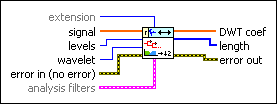extension specifies the method to use to pad data at the borders of the input signal. The extension length is equal to the length of the wavelet filters. When you select the extension method, make the transition between the input signal and the padded data as smooth as possible because a smooth transition generates fewer large detail coefficients and enhances the efficiency of the signal representation.

 0 Zero padding—Uses zeros to pad the input data. Watch for abrupt transitions between the padded zeros and the input data, which causes large artifacts near the transition. 1 Symmetric (default)—Uses replications of the input data to pad the data, except that this VI left-flips the block at the input and right-flips the block at the end. 2 Periodic—Adds a replication of the input data block before and another replication after the input data block to pad the data.signal specifies the input signal.levels specifies the number of levels in the discrete wavelet analysis. levels must be a positive integer no greater than log2(Ls), where Ls is the length of the 1D signal or the minimum dimensional size of the 2D signal. Otherwise, you can set the value to –1, which indicates that this VI sets levels as the largest integer no greater than log2(Ls). The default is –1.wavelet specifies the wavelet type to use for the discrete wavelet analysis. The default is db02. The options include two types: orthogonal (Haar, Daubechies (dbxx), Coiflets (coifx), Symmlets (symx)) and biorthogonal (Biorthogonal (biorx_x), including FBI (bior4_4 (FBI))), where x indicates the order of the wavelet. The higher the order, the smoother the wavelet. The orthogonal wavelets are not redundant and are suitable for signal or image denoising and compression. The biorthogonal wavelets usually have the linear phase property and are suitable for signal or image feature extraction. If you want to use other types of wavelets, do not wire this input. Instead, use the Wavelet Design Express VI to design the wavelet you want and wire the resulting analysis filters to the analysis filters input.error in describes error conditions that occur before this node runs. This input provides standard error in functionality.analysis filters specifies the coefficients of the lowpass analysis filters and the highpass analysis filters for the wavelet you specify. If you specify a value for analysis filters, this VI ignores the settings in the wavelet input. You can use the Wavelet Design Express VI to design the analysis filters and the corresponding synthesis filters.lowpass specifies the coefficients of the lowpass analysis filter, which this VI uses to compute the approximation coefficients.highpass specifies the coefficients of the highpass analysis filter, which this VI uses to compute the detail coefficients.DWT coef returns the approximation coefficients and the detail coefficients from the multi-level discrete wavelet decomposition. This VI concatenates the coefficients into a array starting with the approximation coefficients at the largest level followed by the detail coefficients at all levels in descending order. You can use the WA Get Coefficients of Discrete Wavelet Transform VI to read the approximation coefficients or detail coefficients at a specific level. Wire DWT coef to the DWT coef input of the WA Get Coefficients of Discrete Wavelet Transform VI.length returns, in a 1D array, the number of approximation and detail coefficients at each level. At a decomposition level of N, length is equal to N+2. The first element of length always is equal to the number of approximation coefficients. The last element of length indicates the total number of raw data samples. The length of the detail coefficients is arranged in descending order. You can use the WA Get Coefficients of Discrete Wavelet Transform VI to read the approximation coefficients or detail coefficients at a specific level. Wire length to the length input of the WA Get Coefficients of Discrete Wavelet Transform VI.error out contains error information. This output provides standard error out functionality.

## WA 2D Discrete Wavelet Transform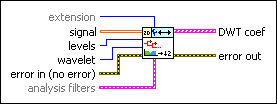extension specifies the method to use to pad data at the borders of the input signal. The extension length is equal to the length of the wavelet filters. When you select the extension method, make the transition between the input signal and the padded data as smooth as possible because a smooth transition generates fewer large detail coefficients and enhances the efficiency of the signal representation.

 0 Zero padding—Uses zeros to pad the input data. Watch for abrupt transitions between the padded zeros and the input data, which causes large artifacts near the transition. 1 Symmetric (default)—Uses replications of the input data to pad the data, except that this VI left-flips the block at the input and right-flips the block at the end. 2 Periodic—Adds a replication of the input data block before and another replication after the input data block to pad the data.signal specifies the 2D input signal.levels specifies the number of levels in the discrete wavelet analysis. levels must be a positive integer no greater than log2(Ls), where Ls is the length of the 1D signal or the minimum dimensional size of the 2D signal. Otherwise, you can set the value to –1, which indicates that this VI sets levels as the largest integer no greater than log2(Ls). The default is –1.wavelet specifies the wavelet type to use for the discrete wavelet analysis. The default is db02. The options include two types: orthogonal (Haar, Daubechies (dbxx), Coiflets (coifx), Symmlets (symx)) and biorthogonal (Biorthogonal (biorx_x), including FBI (bior4_4 (FBI))), where x indicates the order of the wavelet. The higher the order, the smoother the wavelet. The orthogonal wavelets are not redundant and are suitable for signal or image denoising and compression. The biorthogonal wavelets usually have the linear phase property and are suitable for signal or image feature extraction. If you want to use other types of wavelets, do not wire this input. Instead, use the Wavelet Design Express VI to design the wavelet you want and wire the resulting analysis filters to the analysis filters input.error in describes error conditions that occur before this node runs. This input provides standard error in functionality.analysis filters specifies the coefficients of the lowpass analysis filters and the highpass analysis filters for the wavelet you specify. If you specify a value for analysis filters, this VI ignores the settings in the wavelet input. You can use the Wavelet Design Express VI to design the analysis filters and the corresponding synthesis filters.lowpass specifies the coefficients of the lowpass analysis filter, which this VI uses to compute the approximation coefficients.highpass specifies the coefficients of the highpass analysis filter, which this VI uses to compute the detail coefficients.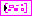DWT coef returns the approximation coefficients and the detail coefficients from the multi-level discrete wavelet transform (DWT). Each element of the array contains the 2D DWT results of one level. The ith element stores the approximation coefficients and the detail coefficients at level i+1. You can use the WA Get Coefficients of Discrete Wavelet Transform VI to read the approximation coefficients or detail coefficients at a specific level. Wire DWT coef to the DWT coef input of the WA Get Coefficients of Discrete Wavelet Transform VI.low_low returns the approximation coefficients from the lowpass analysis filtering on each row and each column. The low_low coefficients are a low-resolution approximation of the original 2D signal.low_high returns the detail coefficients from the lowpass analysis filtering on each row and the highpass analysis filtering on each column. The high-frequency signal along the column direction influences the low_high coefficients.high_low returns the detail coefficients from the highpass analysis filtering on each row and the lowpass analysis filtering on each column. The high-frequency signal along the row direction influences the high_low coefficients.high_high returns the detail coefficients from the highpass analysis filtering on each row and each column. The high-frequency signal along the diagonal direction influences the high_high coefficients.error out contains error information. This output provides standard error out functionality.

## WA Discrete Wavelet Transform Details

The following illustration shows an example of a three-level discrete wavelet decomposition, where you set the levels input of this VI to 3. The length of the input signal is 16 points.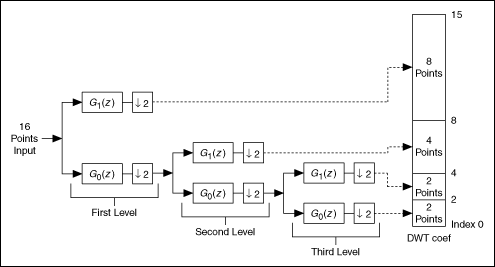G1(z) denotes that the signal passes through a highpass filter. G0(z) denotes that the signal passes through a lowpass filter. G1(z) and G0(z) form the analysis filter bank.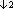denotes a decimation on the signal with a factor of 2.

Using information in the previous illustration, you can see that the DWT coef output contains the approximation coefficients of the largest level and the details coefficients of each level. You also can see that the length output of this VI contains the following elements, where the last element is the length of the input signal: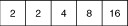## 2D Discrete Wavelet Transform

You easily can extend the 1D discrete wavelet decomposition and reconstruction to 2D signal processing. At each level, this VI implements the 1D DWT on each row signal. Then, this VI applies the 1D DWT to each column of the preceding output. This VI implements the inverse transform with the reverse operation. The following illustration shows the filter bank implementation for a 2D DWT.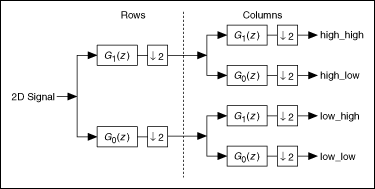low_low is the approximation of the input 2D signal at a larger scale. low_high, high_low, and high_high correspond to the detail information along the column, row, and diagonal directions.

The following illustrations show an image fed into the 2D DWT and the resulting DWT coef.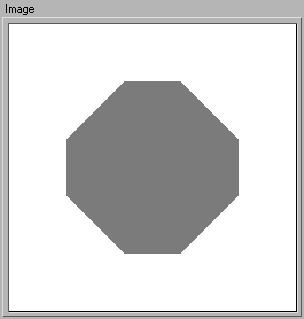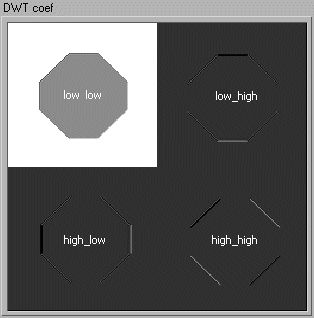Notice that the maxima (white points) and the minima (black points) of low_high are located around the column edges, the maxima and the minima of high_low are near the row edges, and the maxima and the minima of high_high are near the diagonal edges. Refer to A Wavelet Tour of Signal Processing for more information about the DWT.

## Examples

Refer to the following VIs for examples of using the WA Discrete Wavelet Transform VI:

• Image Compression VI: labview\examples\Wavelet Analysis\WAApplications
• Get and Set Single Level Detail Coefficients VI: labview\examples\Wavelet Analysis\WAGettingStarted
• Wavelet Transform and Reconstruction with Image VI: labview\examples\Wavelet Analysis\WAGettingStarted
• Piece Polynomial Function Approx and Comp VI: labview\examples\Wavelet Analysis\WAGettingStarted
• Multiscale Analysis VI: labview\examples\Wavelet Analysis\WAApplications
• Get and Set Approximation Coefficients VI: labview\examples\Wavelet Analysis\WAGettingStarted
• ECG Compression VI: labview\examples\Wavelet Analysis\WAApplications